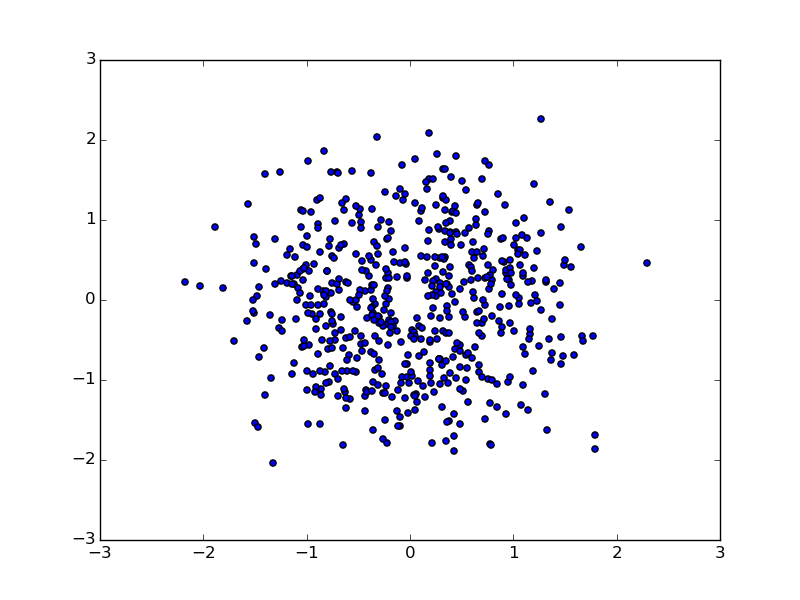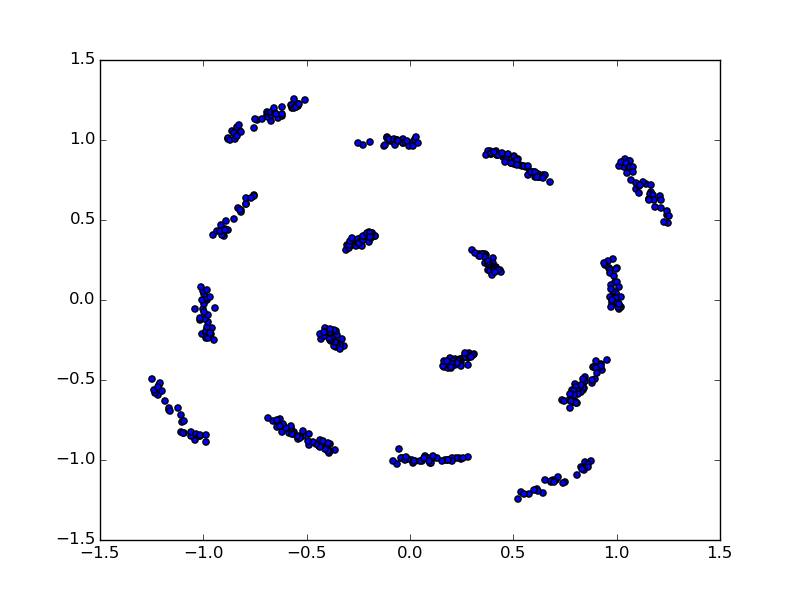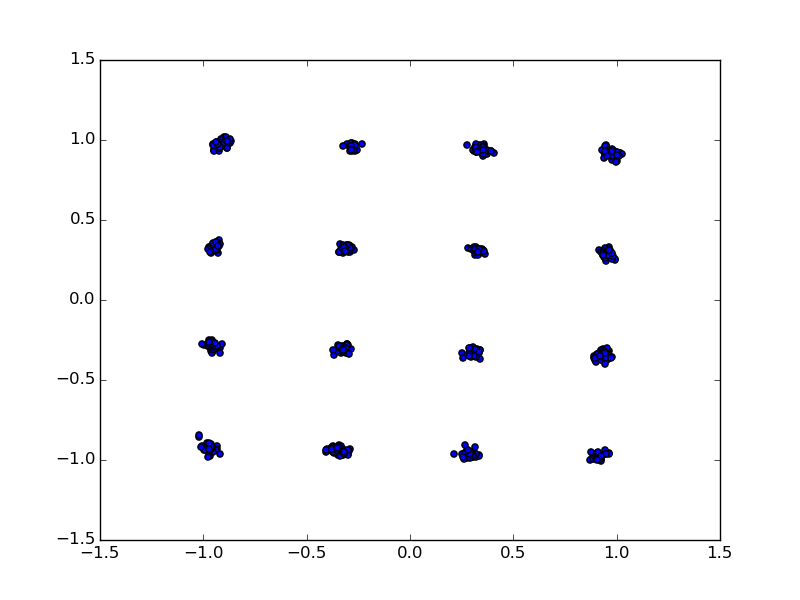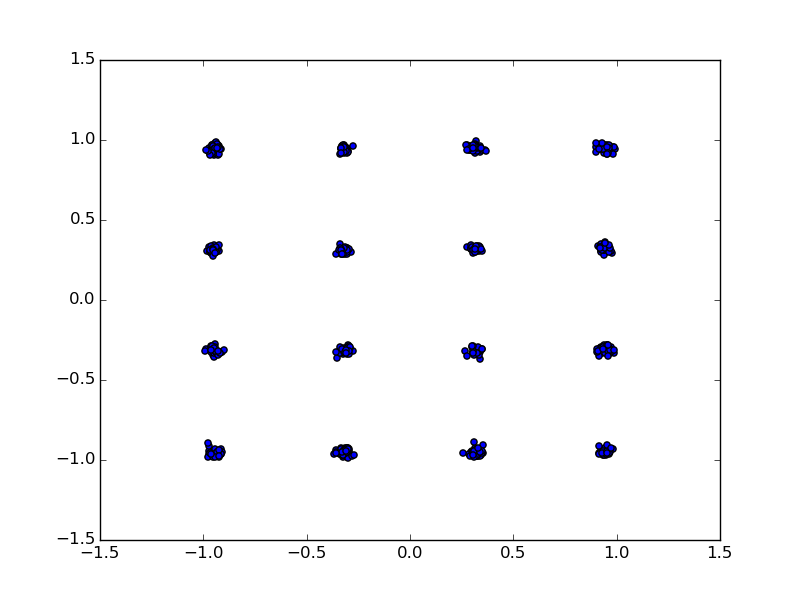# Frequency Offset Correction¶

This paper  explains why frequency offset occurs and how to correct it. In a nutshell, there are two types of frequency offsets. The first is called Carrier Frequency Offset (CFO) and is caused by the difference between the transmitter and receiver’s Local Oscillator (LO). This symptom of this offset is a phase rotation of incoming I/Q samples (time domain). The second is Sampling Frequency Offset (SFO) and is caused by the sampling effect. The symptom of this offset is a phase rotation of constellation points after FFT (frequency domain).

The CFO can be corrected with the help of short preamble (Coarse) long preamble (Fine). And the SFO can be corrected using the pilot sub-carriers in each OFDM symbol. Before we get into how exactly the correction is done. Let’s see visually how each correction step helps in the final constellation plane.Fig. 5 Constellation Points Without Any CorrectionFig. 6 Constellation Points With Only Coarse CorrectionFig. 7 Constellation Points With both Coarse and Fine CorrectionFig. 8 Constellation Points With Coarse, Fine and Pilot Correction

Fig. 5 to Fig. 8 shows the constellation points of a 16-QAM modulated 802.11a packet.

## Coarse CFO Correction¶

The coarse CFO can be estimated using the short preamble as follows:

(1)$\alpha_{ST} = \frac{1}{16}\angle(\sum_{i=0}^{N-1}\overline{S[i]}S[i+16])$

where $$\angle(\cdot)$$ is the phase of complex number and $$N \le 144 (160 - 16)$$ is the subset of short preambles utilized. The intuition is that the phase difference between S[i] and S[i+16] represents the accumulated CFO over 16 samples.

After getting $$\alpha_{ST}$$, each following I/Q samples (starting from long preamble) are corrected as:

(2)$S'[m] = S[m]e^{-jm\alpha_{ST}}, m = 0, 1, 2, \ldots$

In OpenOFDM, the coarse CFO is calculated in the sync_short module, and we set $$N=64$$. The prod_avg in Fig. 4 is fed into a moving_avg module with window size set to 64.

## Fine CFO Correction¶

A finer estimation of the CFO can be obtained with the help of long training sequence inside the long preamble.

The long preamble contains two identify training sequence (64 samples each at 20 MSPS), the phase offset can be calculated as:

(3)$\alpha_{LT} = \frac{1}{64}\angle(\sum_{i=0}^{63}\overline{S[i]}S[i+64])$

This step is omitted in OpenOFDM due to the limited resolution of phase estimation and rotation in the look up table.

  Sourour, Essam, Hussein El-Ghoroury, and Dale McNeill. “Frequency Offset Estimation and Correction in the IEEE 802.11 a WLAN.” Vehicular Technology Conference, 2004. VTC2004-Fall. 2004 IEEE 60th. Vol. 7. IEEE, 2004.